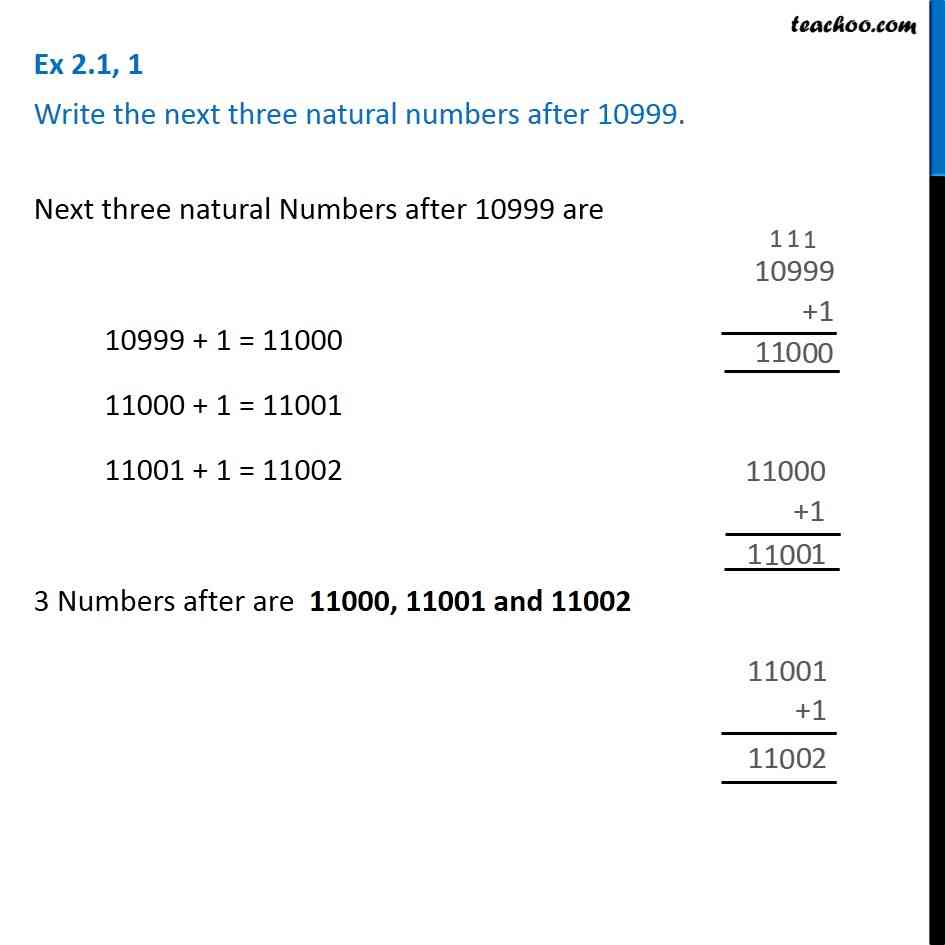1. Chapter 2 Class 6 Whole Numbers
2. Concept wise
3. Definition

Transcript

Ex 2.1, 1 - Chapter 2 Class 6 Whole Numbers - NCERT Solutions Write the next three natural numbers after 10999 Next three natural Numbers after 10999 are 10999 + 1 = 11000 11000 + 1 = 11001 11001 + 1 = 11002 3 Numbers after are 11000, 11001 and 11002

Definition